Search by Topic

Resources tagged with Factors and multiples similar to Light the Lights:

Filter by: Content type:
Age range:
Challenge level:

There are 112 results

Broad Topics > Numbers and the Number System > Factors and multiplesLight the Lights

Age 5 to 7 Challenge Level:

Investigate which numbers make these lights come on. What is the smallest number you can find that lights up all the lights?Venn Diagrams

Age 5 to 11 Challenge Level:

Use the interactivities to complete these Venn diagrams.One of Thirty-six

Age 5 to 7 Challenge Level:

Can you find the chosen number from the grid using the clues?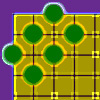Making Shapes

Age 5 to 7 Challenge Level:

Arrange any number of counters from these 18 on the grid to make a rectangle. What numbers of counters make rectangles? How many different rectangles can you make with each number of counters?Seven Flipped

Age 7 to 11 Challenge Level:

Investigate the smallest number of moves it takes to turn these mats upside-down if you can only turn exactly three at a time.Light the Lights Again

Age 7 to 11 Challenge Level:

Each light in this interactivity turns on according to a rule. What happens when you enter different numbers? Can you find the smallest number that lights up all four lights?I Like ...

Age 5 to 7 Challenge Level:

Mr Gilderdale is playing a game with his class. What rule might he have chosen? How would you test your idea?Curious Number

Age 7 to 11 Challenge Level:

Can you order the digits from 1-3 to make a number which is divisible by 3 so when the last digit is removed it becomes a 2-figure number divisible by 2, and so on?Growing Garlic

Age 5 to 7 Challenge Level:

Ben and his mum are planting garlic. Use the interactivity to help you find out how many cloves of garlic they might have had.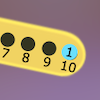Are You Well Balanced?

Age 5 to 7 Challenge Level:

Can you work out how to balance this equaliser? You can put more than one weight on a hook.Multiplication Square Jigsaw

Age 7 to 11 Challenge Level:

Can you complete this jigsaw of the multiplication square?Number Detective

Age 5 to 11 Challenge Level:

Follow the clues to find the mystery number.Skip Counting

Age 5 to 7 Challenge Level:

Find the squares that Froggie skips onto to get to the pumpkin patch. She starts on 3 and finishes on 30, but she lands only on a square that has a number 3 more than the square she skips from.Grouping Goodies

Age 5 to 7 Challenge Level:

Pat counts her sweets in different groups and both times she has some left over. How many sweets could she have had?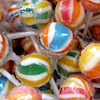Lots of Lollies

Age 5 to 7 Challenge Level:

Frances and Rishi were given a bag of lollies. They shared them out evenly and had one left over. How many lollies could there have been in the bag?The Set of Numbers

Age 5 to 7 Challenge Level:

Can you place the numbers from 1 to 10 in the grid?Share Bears

Age 5 to 7 Challenge Level:

Yasmin and Zach have some bears to share. Which numbers of bears can they share so that there are none left over?Down to Nothing

Age 7 to 11 Challenge Level:

A game for 2 or more people. Starting with 100, subratct a number from 1 to 9 from the total. You score for making an odd number, a number ending in 0 or a multiple of 6.Odds and Threes

Age 7 to 11 Challenge Level:

A game for 2 people using a pack of cards Turn over 2 cards and try to make an odd number or a multiple of 3.Same Length Trains

Age 5 to 7 Challenge Level:

How many trains can you make which are the same length as Matt's, using rods that are identical?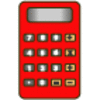Constant Counting

Age 5 to 7 Challenge Level:

You can make a calculator count for you by any number you choose. You can count by ones to reach 24. You can count by twos to reach 24. What else can you count by to reach 24?Age 7 to 11 Challenge Level:

If you have only four weights, where could you place them in order to balance this equaliser?Factor Lines

Age 7 to 14 Challenge Level:

Arrange the four number cards on the grid, according to the rules, to make a diagonal, vertical or horizontal line.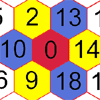Nineteen Hexagons

Age 5 to 7 Challenge Level:

In this maze of hexagons, you start in the centre at 0. The next hexagon must be a multiple of 2 and the next a multiple of 5. What are the possible paths you could take?Which Numbers? (1)

Age 7 to 11 Challenge Level:

I am thinking of three sets of numbers less than 101. They are the red set, the green set and the blue set. Can you find all the numbers in the sets from these clues?Times Tables Shifts

Age 7 to 11 Challenge Level:

In this activity, the computer chooses a times table and shifts it. Can you work out the table and the shift each time?Tables Teaser

Age 5 to 7 Challenge Level:

How will you work out which numbers have been used to create this multiplication square?Which Numbers? (2)

Age 7 to 11 Challenge Level:

I am thinking of three sets of numbers less than 101. Can you find all the numbers in each set from these clues?Colour Wheels

Age 7 to 11 Challenge Level:

Imagine a wheel with different markings painted on it at regular intervals. Can you predict the colour of the 18th mark? The 100th mark?Cuisenaire Environment

Age 5 to 11 Challenge Level:

An environment which simulates working with Cuisenaire rods.Multiples Grid

Age 7 to 11 Challenge Level:

What do the numbers shaded in blue on this hundred square have in common? What do you notice about the pink numbers? How about the shaded numbers in the other squares?Multiply Multiples 1

Age 7 to 11 Challenge Level:

Can you complete this calculation by filling in the missing numbers? In how many different ways can you do it?Multiply Multiples 2

Age 7 to 11 Challenge Level:

Can you work out some different ways to balance this equation?Multiply Multiples 3

Age 7 to 11 Challenge Level:

Have a go at balancing this equation. Can you find different ways of doing it?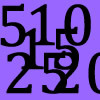Doubling Fives

Age 5 to 7 Challenge Level:

This activity focuses on doubling multiples of five.Clapping Times

Age 5 to 7 Challenge Level:

If you count from 1 to 20 and clap more loudly on the numbers in the two times table, as well as saying those numbers loudly, which numbers will be loud?Give Me Four Clues

Age 7 to 11 Challenge Level:

Four of these clues are needed to find the chosen number on this grid and four are true but do nothing to help in finding the number. Can you sort out the clues and find the number?Number Tracks

Age 7 to 11 Challenge Level:

Ben’s class were cutting up number tracks. First they cut them into twos and added up the numbers on each piece. What patterns could they see?It Figures

Age 7 to 11 Challenge Level:

Suppose we allow ourselves to use three numbers less than 10 and multiply them together. How many different products can you find? How do you know you've got them all?What Do You Need?

Age 7 to 11 Challenge Level:

Four of these clues are needed to find the chosen number on this grid and four are true but do nothing to help in finding the number. Can you sort out the clues and find the number?Sorting Numbers

Age 5 to 7 Challenge Level:

Can you sort numbers into sets? Can you give each set a name?Got It

Age 7 to 14 Challenge Level:

A game for two people, or play online. Given a target number, say 23, and a range of numbers to choose from, say 1-4, players take it in turns to add to the running total to hit their target.Money Measure

Age 7 to 11 Challenge Level:

How can you use just one weighing to find out which box contains the lighter ten coins out of the ten boxes?Multiplication Series: Number Arrays

Age 5 to 11

This article for teachers describes how number arrays can be a useful representation for many number concepts.Crossings

Age 7 to 11 Challenge Level:

In this problem we are looking at sets of parallel sticks that cross each other. What is the least number of crossings you can make? And the greatest?Tiling

Age 7 to 11 Challenge Level:

An investigation that gives you the opportunity to make and justify predictions.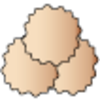Lots of Biscuits!

Age 5 to 7 Challenge Level:

Help share out the biscuits the children have made.Table Patterns Go Wild!

Age 7 to 11 Challenge Level:

Nearly all of us have made table patterns on hundred squares, that is 10 by 10 grids. This problem looks at the patterns on differently sized square grids.Becky's Number Plumber

Age 7 to 11 Challenge Level:

Becky created a number plumber which multiplies by 5 and subtracts 4. What do you notice about the numbers that it produces? Can you explain your findings?Numbers as Shapes

Age 5 to 7 Challenge Level:

Use cubes to continue making the numbers from 7 to 20. Are they sticks, rectangles or squares?